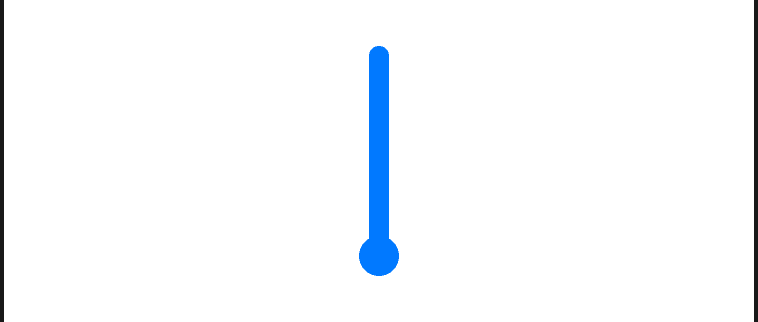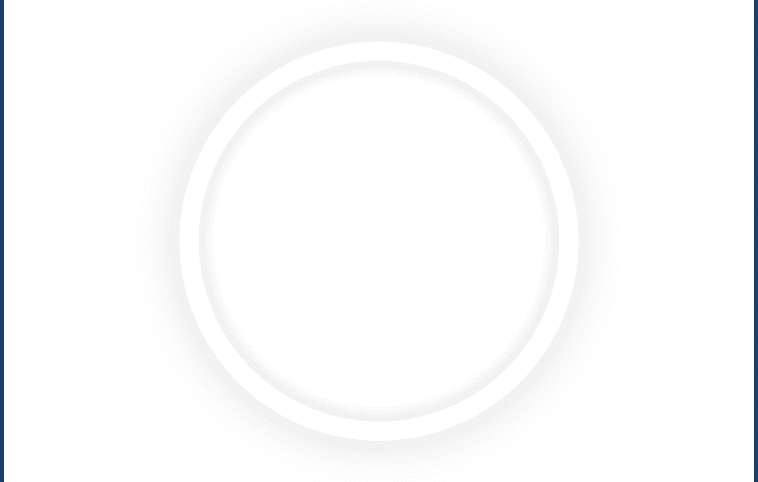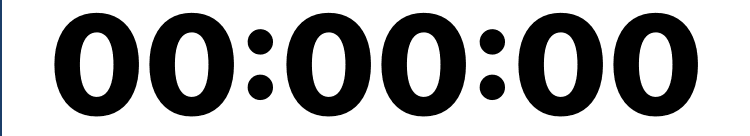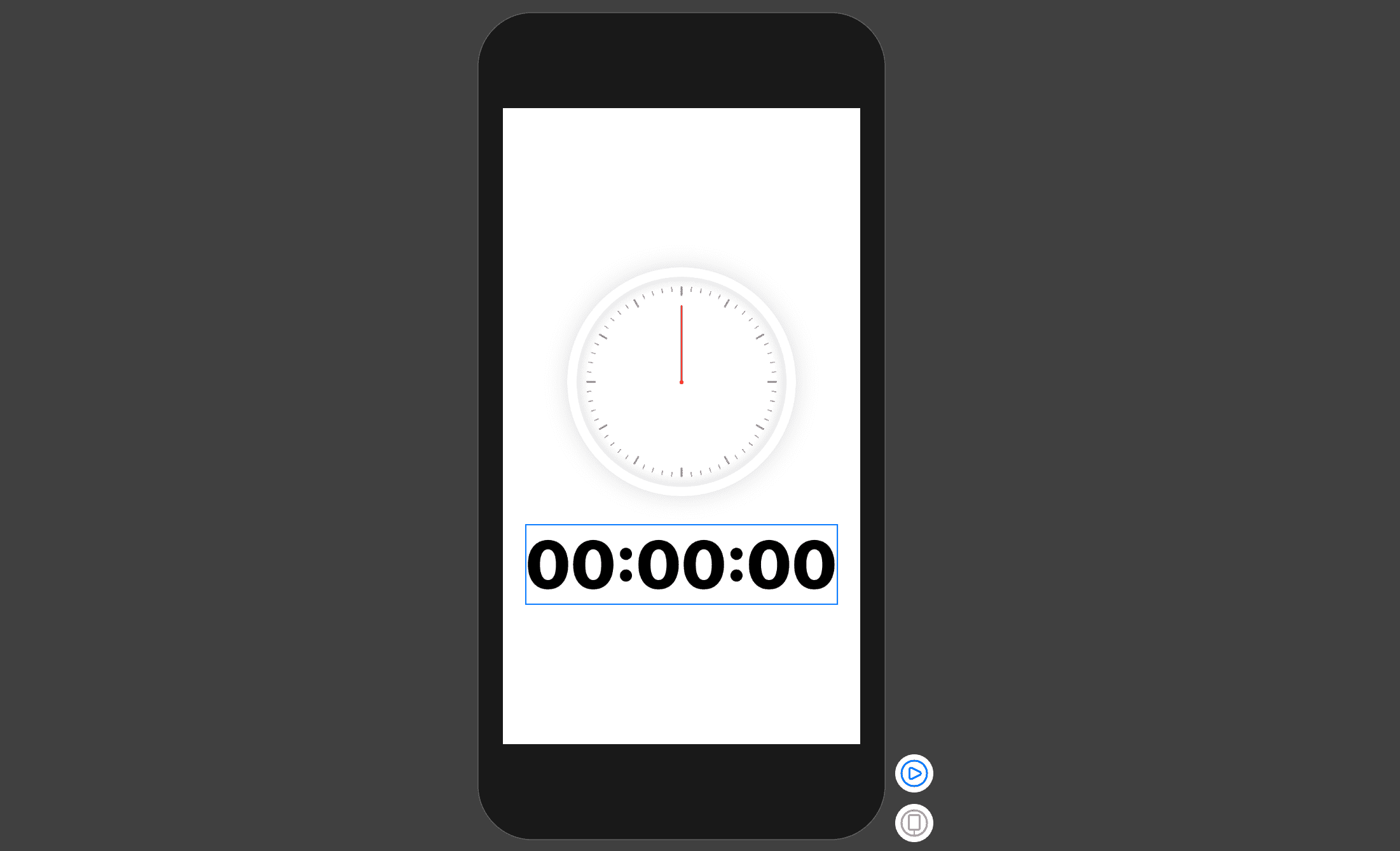# Analogue watch design

Thursday, 14 November 2019
Updated 2 years ago
I will start sending email newsletters once in a while, so in order to get notified when I release new content, make sure to follow me on twitter @liquidcoder .

## Introduction

Today we are going to design and create an analogue watch in swiftUI. This will be a small piece from an upcoming project I am working on, it’s a simple app. Writing about the entire thing might be tiresome for readers and me, so I will divide this into smaller pieces. From now on I will be writing about pieces of a project until the entire thing is fully put together.

With that out of the way, let’s get down to business.

## Requirements

• Xcode 12 or greater
• MacOS Catalina or greater
• Swift 5.0 or greater
• iOS 14 or greater

## Get started

• Open Xcode
• Create a new Xcode project
• Select single view app and click next
• Name your app and make sure the user interface is Swift UI
• Last, click Finish.
• Before we start, create a folder named Views. This is where we will be keeping our views. Let’s get started.

## Watch Hand

Inside the Views folder, add a new file called WatchHand and inside that file replace everything inside with the following:

``````import SwiftUI

struct WatchHand: View {
var handWidth: CGFloat = 10
var handHeight: CGFloat = 100
var color: Color = Color.blue

var body: some View {
VStack(spacing: -(handWidth / 2)) {
Capsule().frame(width: handWidth, height: handHeight).foregroundColor(color)
Circle().frame(width: handWidth * 2, height: handWidth * 2).foregroundColor(color)
}
}
}

struct WatchHand_Previews: PreviewProvider {
static var previews: some View {
WatchHand()
}
}

``````

The above code stacks a capsule and circle with a negative spacing to create a watch hand design.

Preview## Watch Frame

Create a new file named WatchFrame and replace everything inside with the following:

``````import SwiftUI

struct WatchFrame: View {

var size: CGFloat = 200
var borderSize: CGFloat = 20

var body: some View {

ZStack {
Circle()
.frame(width: size, height: size)
.foregroundColor(Color.clear)

Circle().stroke(style: StrokeStyle(lineWidth: borderSize))
.frame(width: size, height: size)
.foregroundColor(Color.white)

}
}

struct WatchFrame_Previews: PreviewProvider {
static var previews: some View {
WatchFrame()
}
}

``````

Notice how we stack 2 circles on the Z axis. The first circle creates an inner shadow by adding a `Gradient` modifier to it and the second creates a border. You can play with the `startRadius` value to find what you like if you don’t like mine.

Preview## Watch View

This part will bring the 2 views together to create our analogue watch. Same as before, creates a new file named WatchView. At the top of the file add these properties:

``````    var diameter: CGFloat = 200
var smallCapsuleHeight: CGFloat = 5
var bigCapsuleHeight: CGFloat = 10

@State var hours: Double = 0.0
@State var minutes: Double = 0.0
@State var seconds: Double = 0.0

``````

Then replace what’s inside the body block with the following:

``````VStack {
Text("\(hours < 10 ? "0" : "")\(Int(hours)):\(minutes < 10 ? "0" : "")\(Int(minutes)):\(seconds < 10 ? "0" : "")\(Int(seconds))")
.font(.system(size: 70, weight: .black))
}
``````Below the `body` block, add this function:

``````    func createWatch() -> some View {

let angle = 360 / 60
let hourAngle = 360 / 12
let interval = 360 / angle

let hourLength: CGFloat =  diameter / 2 * 0.4
let minuteLength: CGFloat = diameter / 2 * 0.7
let secondLength: CGFloat = diameter / 2 * 0.8

return  ZStack {
WatchFrame(size: self.diameter + 40, borderSize: 20)

ForEach(0..<interval){ i in
self.createSecondMark(position: i, angle: angle)
}

WatchHand(handWidth: 2, handHeight: hourLength, color: Color.black)
.offset( y:  -(hourLength / 2) + 1.0)
.rotationEffect(Angle(degrees: hours * Double(hourAngle) + (Double(hourAngle) * minutes / 60.0) ) , anchor: .center)

WatchHand(handWidth: 2, handHeight: minuteLength, color: Color.black)
.offset( y:  -(minuteLength / 2) + 1.0)
.rotationEffect(Angle(degrees: minutes * Double(angle) + (Double(angle) * seconds / 60.0)) , anchor: .center)

WatchHand(handWidth: 2, handHeight: secondLength, color: Color.red)
.offset( y:  -(secondLength / 2) + 1.0)
.rotationEffect(Angle(degrees: seconds * Double(angle)) , anchor: .center)
}
}
``````

The above code does the following:

1. Calculates the angle between 2 second marks by dividing the entire circle by 60 which is obviously the number of seconds and we will use the same value for minutes, as for hours, we divide the entire circle by 12.
2. We put everything in a ZStack container because we want them to be stacked on top of each other. First we add the frame, then we create the second marks. You will get an error because the `createSecondmark` does not exist yet. Last we add the 3 hands (hour, minute and second).

Below the above function, add the following:

``````
func createSecondMarks(position: Int, angle: Int) -> some View{
if position.isMultiple(of: 5) {
return Capsule().frame(width:  2, height:  self.bigCapsuleHeight).offset(y: self.diameter / 2 - self.bigCapsuleHeight / 2).rotationEffect(Angle(degrees: Double(position * angle) )).foregroundColor(Color.gray)
} else {
return Capsule().frame(width:  1 , height: self.smallCapsuleHeight).offset(y: self.diameter / 2 - self.smallCapsuleHeight / 2).rotationEffect(Angle(degrees: Double(position * angle) )).foregroundColor(Color.gray)
}
}

``````

The above code create the second marks and makes the hour marks a bit thicker and longer than the normal marks. Here the order of modifiers is very important, make sure you write exactly the same code. The code does the following:

1. First give the capsule a frame.
2. Then push it to the edge of the circle by setting its y offset to half the diameter minus its length.
3. And last I set its rotation effect where the angle is the position times the angle we calculated above. Now call the `createWatch()` in the `body` block, above the `Text()` like this:
``````VStack {
createWatch()
Text("\(hours < 10 ? "0" : "")\(Int(hours)):\(minutes < 10 ? "0" : "")\(Int(minutes)):\(seconds < 10 ? "0" : "")\(Int(seconds))")
.font(.system(size: 70, weight: .black))
}
``````

PreviewThe UI Part is done and ready. Let’s now work on the logic part.

## Time Logic

Below the `createSecondMarks` function, add the following code:

``````    func getTimeComponent(from date: Date, format: String) -> Double {
let formatter = DateFormatter()
formatter.dateFormat = format
formatter.locale = Locale.autoupdatingCurrent
let component = formatter.string(from: date)

if let value = Double(component) {
return value
}
return 0.0
}
``````

This function formats and returns a date component in Double, we will use it to retrieve hours, minutes and seconds from a particular date.

Then add the following modifier to the `VStack` container in the `body` block:

``````.onAppear{
let date = Date()
self.hours = self.getTimeComponent(from: date, format: "HH")
self.minutes = self.getTimeComponent(from: date, format: "mm")
self.seconds = self.getTimeComponent(from: date, format: "ss")

Timer.scheduledTimer(withTimeInterval: 1, repeats: true) { timer in
let date = timer.fireDate

self.hours = self.getTimeComponent(from: date, format: "HH")
self.minutes = self.getTimeComponent(from: date, format: "mm")
self.seconds = self.getTimeComponent(from: date, format: "ss")
}
}
``````

The code inside onAppear will run every time the `VStack` first appears and does the following:

1. First get the current date and updates the hands.
2. Then schedules a timer which will be running every second to update the time and move the hands. Before running the app, make sure you call the `WatchView` inside the `ContentView`’s body block like this:
`````` var body: some View {
WatchView()
}
``````